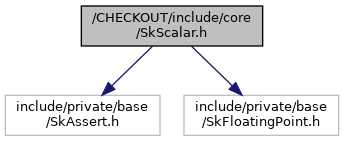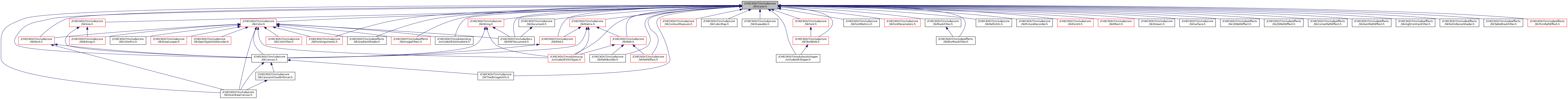Skia 2D Graphics Library
SkScalar.h File Reference
`#include "include/private/SkFloatingPoint.h"`
Include dependency graph for SkScalar.h:This graph shows which files directly or indirectly include this file:Go to the source code of this file.

## Macros

#define SK_SCALAR_IS_FLOAT   1

#define SK_Scalar1   1.0f

#define SK_ScalarHalf   0.5f

#define SK_ScalarSqrt2   SK_FloatSqrt2

#define SK_ScalarPI   SK_FloatPI

#define SK_ScalarTanPIOver8   0.414213562f

#define SK_ScalarRoot2Over2   0.707106781f

#define SK_ScalarMax   3.402823466e+38f

#define SK_ScalarInfinity   SK_FloatInfinity

#define SK_ScalarNegativeInfinity   SK_FloatNegativeInfinity

#define SK_ScalarNaN   SK_FloatNaN

#define SkScalarFloorToScalar(x)   sk_float_floor(x)

#define SkScalarCeilToScalar(x)   sk_float_ceil(x)

#define SkScalarRoundToScalar(x)   sk_float_floor((x) + 0.5f)

#define SkScalarTruncToScalar(x)   sk_float_trunc(x)

#define SkScalarFloorToInt(x)   sk_float_floor2int(x)

#define SkScalarCeilToInt(x)   sk_float_ceil2int(x)

#define SkScalarRoundToInt(x)   sk_float_round2int(x)

#define SkScalarAbs(x)   sk_float_abs(x)

#define SkScalarCopySign(x, y)   sk_float_copysign(x, y)

#define SkScalarMod(x, y)   sk_float_mod(x,y)

#define SkScalarSqrt(x)   sk_float_sqrt(x)

#define SkScalarPow(b, e)   sk_float_pow(b, e)

#define SkScalarSin(radians)   (float)sk_float_sin(radians)

#define SkScalarCos(radians)   (float)sk_float_cos(radians)

#define SkScalarTan(radians)   (float)sk_float_tan(radians)

#define SkScalarASin(val)   (float)sk_float_asin(val)

#define SkScalarACos(val)   (float)sk_float_acos(val)

#define SkScalarATan2(y, x)   (float)sk_float_atan2(y,x)

#define SkScalarExp(x)   (float)sk_float_exp(x)

#define SkScalarLog(x)   (float)sk_float_log(x)

#define SkScalarLog2(x)   (float)sk_float_log2(x)

#define SkIntToScalar(x)   static_cast<SkScalar>(x)

#define SkIntToFloat(x)   static_cast<float>(x)

#define SkScalarTruncToInt(x)   sk_float_saturate2int(x)

#define SkScalarToFloat(x)   static_cast<float>(x)

#define SkFloatToScalar(x)   static_cast<SkScalar>(x)

#define SkScalarToDouble(x)   static_cast<double>(x)

#define SkDoubleToScalar(x)   sk_double_to_float(x)

#define SK_ScalarMin   (-SK_ScalarMax)

#define SkScalarInvert(x)   sk_ieee_float_divide_TODO_IS_DIVIDE_BY_ZERO_SAFE_HERE(SK_Scalar1, (x))

#define SkScalarAve(a, b)   (((a) + (b)) * SK_ScalarHalf)

#define SkScalarHalf(a)   ((a) * SK_ScalarHalf)

#define SkDegreesToRadians(degrees)   ((degrees) * (SK_ScalarPI / 180))

#define SkRadiansToDegrees(radians)   ((radians) * (180 / SK_ScalarPI))

#define SK_ScalarNearlyZero   (SK_Scalar1 / (1 << 12))

## Typedefs

typedef float SkScalar

## Functions

static bool SkScalarIsNaN (SkScalar x)

static bool SkScalarIsFinite (SkScalar x)
Returns true if x is not NaN and not infinite. More...

static bool SkScalarsAreFinite (SkScalar a, SkScalar b)

static bool SkScalarsAreFinite (const SkScalar array[], int count)

static int SkDScalarRoundToInt (SkScalar x)
Variant of SkScalarRoundToInt, that performs the rounding step (adding 0.5) explicitly using double, to avoid possibly losing the low bit(s) of the answer before calling floor(). More...

static SkScalar SkScalarFraction (SkScalar x)
Returns the fractional part of the scalar. More...

static SkScalar SkScalarSquare (SkScalar x)

static bool SkScalarIsInt (SkScalar x)

static int SkScalarSignAsInt (SkScalar x)
Returns -1 || 0 || 1 depending on the sign of value: -1 if x < 0 0 if x == 0 1 if x > 0. More...

static SkScalar SkScalarSignAsScalar (SkScalar x)

static bool SkScalarNearlyZero (SkScalar x, SkScalar tolerance=SK_ScalarNearlyZero)

static bool SkScalarNearlyEqual (SkScalar x, SkScalar y, SkScalar tolerance=SK_ScalarNearlyZero)

static float SkScalarSinSnapToZero (SkScalar radians)

static float SkScalarCosSnapToZero (SkScalar radians)

static SkScalar SkScalarInterp (SkScalar A, SkScalar B, SkScalar t)
Linearly interpolate between A and B, based on t. More...

SkScalar SkScalarInterpFunc (SkScalar searchKey, const SkScalar keys[], const SkScalar values[], int length)
Interpolate along the function described by (keys[length], values[length]) for the passed searchKey. More...

static bool SkScalarsEqual (const SkScalar a[], const SkScalar b[], int n)

## ◆ SK_Scalar1

 #define SK_Scalar1   1.0f

## ◆ SK_SCALAR_IS_FLOAT

 #define SK_SCALAR_IS_FLOAT   1

## ◆ SK_ScalarHalf

 #define SK_ScalarHalf   0.5f

## ◆ SK_ScalarInfinity

 #define SK_ScalarInfinity   SK_FloatInfinity

## ◆ SK_ScalarMax

 #define SK_ScalarMax   3.402823466e+38f

## ◆ SK_ScalarMin

 #define SK_ScalarMin   (-SK_ScalarMax)

## ◆ SK_ScalarNaN

 #define SK_ScalarNaN   SK_FloatNaN

## ◆ SK_ScalarNearlyZero

 #define SK_ScalarNearlyZero   (SK_Scalar1 / (1 << 12))

## ◆ SK_ScalarNegativeInfinity

 #define SK_ScalarNegativeInfinity   SK_FloatNegativeInfinity

## ◆ SK_ScalarPI

 #define SK_ScalarPI   SK_FloatPI

## ◆ SK_ScalarRoot2Over2

 #define SK_ScalarRoot2Over2   0.707106781f

## ◆ SK_ScalarSqrt2

 #define SK_ScalarSqrt2   SK_FloatSqrt2

## ◆ SK_ScalarTanPIOver8

 #define SK_ScalarTanPIOver8   0.414213562f

## ◆ SkDegreesToRadians

 #define SkDegreesToRadians ( degrees ) ((degrees) * (SK_ScalarPI / 180))

## ◆ SkDoubleToScalar

 #define SkDoubleToScalar ( x ) sk_double_to_float(x)

## ◆ SkFloatToScalar

 #define SkFloatToScalar ( x ) static_cast(x)

## ◆ SkIntToFloat

 #define SkIntToFloat ( x ) static_cast(x)

## ◆ SkIntToScalar

 #define SkIntToScalar ( x ) static_cast(x)

## ◆ SkRadiansToDegrees

 #define SkRadiansToDegrees ( radians ) ((radians) * (180 / SK_ScalarPI))

## ◆ SkScalarAbs

 #define SkScalarAbs ( x ) sk_float_abs(x)

## ◆ SkScalarACos

 #define SkScalarACos ( val ) (float)sk_float_acos(val)

## ◆ SkScalarASin

 #define SkScalarASin ( val ) (float)sk_float_asin(val)

## ◆ SkScalarATan2

 #define SkScalarATan2 ( y, x ) (float)sk_float_atan2(y,x)

## ◆ SkScalarAve

 #define SkScalarAve ( a, b ) (((a) + (b)) * SK_ScalarHalf)

## ◆ SkScalarCeilToInt

 #define SkScalarCeilToInt ( x ) sk_float_ceil2int(x)

## ◆ SkScalarCeilToScalar

 #define SkScalarCeilToScalar ( x ) sk_float_ceil(x)

## ◆ SkScalarCopySign

 #define SkScalarCopySign ( x, y ) sk_float_copysign(x, y)

## ◆ SkScalarCos

 #define SkScalarCos ( radians ) (float)sk_float_cos(radians)

## ◆ SkScalarExp

 #define SkScalarExp ( x ) (float)sk_float_exp(x)

## ◆ SkScalarFloorToInt

 #define SkScalarFloorToInt ( x ) sk_float_floor2int(x)

## ◆ SkScalarFloorToScalar

 #define SkScalarFloorToScalar ( x ) sk_float_floor(x)

## ◆ SkScalarHalf

 #define SkScalarHalf ( a ) ((a) * SK_ScalarHalf)

## ◆ SkScalarInvert

 #define SkScalarInvert ( x ) sk_ieee_float_divide_TODO_IS_DIVIDE_BY_ZERO_SAFE_HERE(SK_Scalar1, (x))

## ◆ SkScalarLog

 #define SkScalarLog ( x ) (float)sk_float_log(x)

## ◆ SkScalarLog2

 #define SkScalarLog2 ( x ) (float)sk_float_log2(x)

## ◆ SkScalarMod

 #define SkScalarMod ( x, y ) sk_float_mod(x,y)

## ◆ SkScalarPow

 #define SkScalarPow ( b, e ) sk_float_pow(b, e)

## ◆ SkScalarRoundToInt

 #define SkScalarRoundToInt ( x ) sk_float_round2int(x)

## ◆ SkScalarRoundToScalar

 #define SkScalarRoundToScalar ( x ) sk_float_floor((x) + 0.5f)

## ◆ SkScalarSin

 #define SkScalarSin ( radians ) (float)sk_float_sin(radians)

## ◆ SkScalarSqrt

 #define SkScalarSqrt ( x ) sk_float_sqrt(x)

## ◆ SkScalarTan

 #define SkScalarTan ( radians ) (float)sk_float_tan(radians)

## ◆ SkScalarToDouble

 #define SkScalarToDouble ( x ) static_cast(x)

## ◆ SkScalarToFloat

 #define SkScalarToFloat ( x ) static_cast(x)

## ◆ SkScalarTruncToInt

 #define SkScalarTruncToInt ( x ) sk_float_saturate2int(x)

## ◆ SkScalarTruncToScalar

 #define SkScalarTruncToScalar ( x ) sk_float_trunc(x)

## ◆ SkScalar

 typedef float SkScalar

## ◆ SkDScalarRoundToInt()

 static int SkDScalarRoundToInt ( SkScalar x )
inlinestatic

Variant of SkScalarRoundToInt, that performs the rounding step (adding 0.5) explicitly using double, to avoid possibly losing the low bit(s) of the answer before calling floor().

This routine will likely be slower than SkScalarRoundToInt(), and should only be used when the extra precision is known to be valuable.

In particular, this catches the following case: SkScalar x = 0.49999997; int ix = SkScalarRoundToInt(x); SkASSERT(0 == ix); // <— fails ix = SkDScalarRoundToInt(x); SkASSERT(0 == ix); // <— succeeds

## ◆ SkScalarCosSnapToZero()

 static float SkScalarCosSnapToZero ( SkScalar radians )
inlinestatic

## ◆ SkScalarFraction()

 static SkScalar SkScalarFraction ( SkScalar x )
inlinestatic

Returns the fractional part of the scalar.

## ◆ SkScalarInterp()

 static SkScalar SkScalarInterp ( SkScalar A, SkScalar B, SkScalar t )
inlinestatic

Linearly interpolate between A and B, based on t.

If t is 0, return A If t is 1, return B else interpolate. t must be [0..SK_Scalar1]

## ◆ SkScalarInterpFunc()

 SkScalar SkScalarInterpFunc ( SkScalar searchKey, const SkScalar keys[], const SkScalar values[], int length )

Interpolate along the function described by (keys[length], values[length]) for the passed searchKey.

SearchKeys outside the range keys-keys[Length] clamp to the min or max value. This function assumes the number of pairs (length) will be small and a linear search is used.

Repeated keys are allowed for discontinuous functions (so long as keys is monotonically increasing). If key is the value of a repeated scalar in keys the first one will be used.

## ◆ SkScalarIsFinite()

 static bool SkScalarIsFinite ( SkScalar x )
inlinestatic

Returns true if x is not NaN and not infinite.

## ◆ SkScalarIsInt()

 static bool SkScalarIsInt ( SkScalar x )
inlinestatic

## ◆ SkScalarIsNaN()

 static bool SkScalarIsNaN ( SkScalar x )
inlinestatic

## ◆ SkScalarNearlyEqual()

 static bool SkScalarNearlyEqual ( SkScalar x, SkScalar y, SkScalar tolerance = `SK_ScalarNearlyZero` )
inlinestatic

## ◆ SkScalarNearlyZero()

 static bool SkScalarNearlyZero ( SkScalar x, SkScalar tolerance = `SK_ScalarNearlyZero` )
inlinestatic

## ◆ SkScalarsAreFinite() [1/2]

 static bool SkScalarsAreFinite ( const SkScalar array[], int count )
inlinestatic

## ◆ SkScalarsAreFinite() [2/2]

 static bool SkScalarsAreFinite ( SkScalar a, SkScalar b )
inlinestatic

## ◆ SkScalarsEqual()

 static bool SkScalarsEqual ( const SkScalar a[], const SkScalar b[], int n )
inlinestatic

## ◆ SkScalarSignAsInt()

 static int SkScalarSignAsInt ( SkScalar x )
inlinestatic

Returns -1 || 0 || 1 depending on the sign of value: -1 if x < 0 0 if x == 0 1 if x > 0.

## ◆ SkScalarSignAsScalar()

 static SkScalar SkScalarSignAsScalar ( SkScalar x )
inlinestatic

## ◆ SkScalarSinSnapToZero()

 static float SkScalarSinSnapToZero ( SkScalar radians )
inlinestatic

## ◆ SkScalarSquare()

 static SkScalar SkScalarSquare ( SkScalar x )
inlinestatic